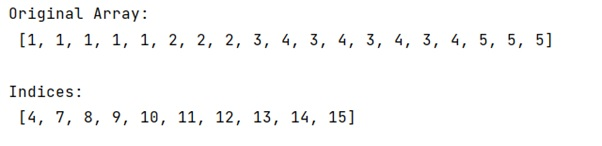# How to find index where elements change value NumPy?

Learn, how to find index where elements change value NumPy in Python?
Submitted by Pranit Sharma, on February 22, 2023

NumPy is an abbreviated form of Numerical Python. It is used for different types of scientific operations in python. Numpy is a vast library in python which is used for almost every kind of scientific or mathematical operation. It is itself an array which is a collection of various methods and functions for processing the arrays.

## NumPy - Finding index where elements change value

Suppose that we are given a NumPy array that contains the same elements, however, there are some different values at certain positions, we need to find an efficient way to know the index where the values of this array change.

For this purpose, we can simply use a for loop over the array and compare each value with its neighbor and return its index if the values do not match.

Let us understand with the help of an example,

## Python code to find index where elements change value NumPy

```# Import numpy
import numpy as np

# Creating a numpy array
arr = [1, 1, 1, 1, 1, 2, 2, 2, 3, 4, 3, 4, 3, 4, 3, 4, 5, 5, 5]

# Display original array
print("Original Array:\n",arr,"\n")

# Finding the indices
l = []
for i in range(len(arr)-1):
if arr[i]!=arr[i+1]:
l.append(i)

# Display indices
print("Indices:\n",l,"\n")
```

Output: